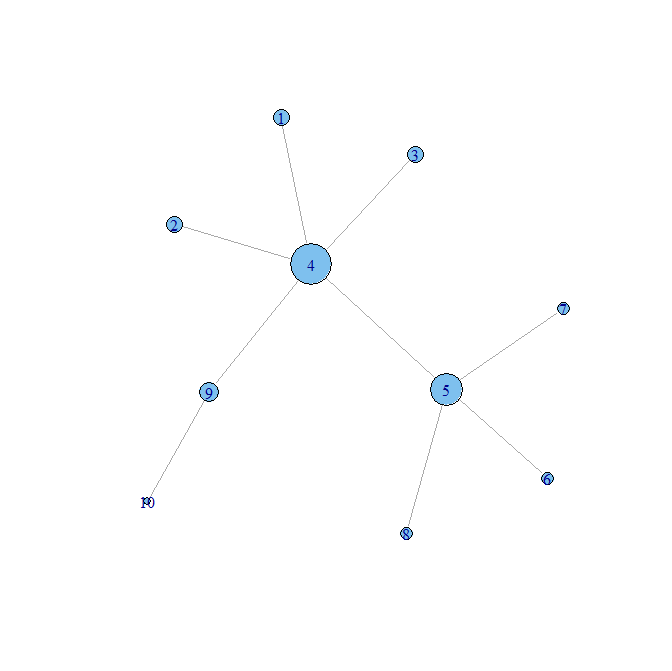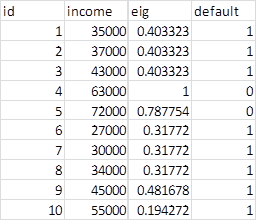## Saturday, April 14, 2012

### Using SNA in Predictive Modeling

In a previous post, I described the basics of social network analysis. I plan to extend that example here with an application in predictive analytics. Let's suppose we have the following network (visualized in R)Suppose we have used the igraph package in R to derive measures of centrality, and we combined that information with other information gathered from our data base, for example income and loan default data. We can then incorporate the centrality measures into a model that predicts default.

Notwithstanding the issues related to linear regression with binary dependent variables, the extremely small sample size, and the fact that this data is totally made up, for illustrative purposes, lets say we have the following data set:A basic linear regression (loan default = B1*eignevector centrality + B2*income) gives the following:

Estimate Std. Error t value Pr(>|t|)
(Intercept)        1.775e+00  1.424e-01  12.464 4.93e-06 ***
creditrisk\$eig    -1.172e+00  2.642e-01  -4.438  0.00302 **
creditrisk\$income -9.807e-06  4.362e-06  -2.249  0.05932 .
---
Signif. codes:  0 ‘***’ 0.001 ‘**’ 0.01 ‘*’ 0.05 ‘.’ 0.1 ‘ ’ 1

Residual standard error: 0.1362 on 7 degrees of freedom
Multiple R-squared: 0.9188,     Adjusted R-squared: 0.8956
F-statistic:  39.6 on 2 and 7 DF,  p-value: 0.0001526

Although the data is made up, we can see that eigenvector centrality is a significant predictor in the regression.  While logistic regression or decision trees may be more appropriate,  I could not obtain results for illustrative purposes using those algorithms, most likely the consequence of the small feature space.

For a couple of actual applications with real data see:

Analysis of Variance Application:

Investigating Student Communities with Network Analysis of Interactions in a Physics Learning Center. Brewe, Eric; Kramer, Laird; O'Brien, George
2009 PHYSICS EDUCATION RESEARCH CONFERENCE. AIP Conference Proceedings, Volume 1179, pp. 105-108 (2009).

Path Analysis:

Ties That Bind: A Social Network Approach to Understanding Student Integration and Persistence
Scott L. Thomas. The Journal of Higher Education , Vol. 71, No. 5 (Sep. - Oct., 2000), pp. 591-615

The R used in this illustration follows:
```# *------------------------------------------------------------------
# | PROGRAM NAME: R_BASIC_SNA
# | DATE: 4/9/12
# | CREATED BY: MATT BOGARD
# | PROJECT FILE: P:\R  Code References\SNA
# *----------------------------------------------------------------
# | PURPOSE: DEMONSTRATION OF BASIC CONCEPTS OF NETWORK ANALYSIS
# | REFERENCES: Conway, Drew. Social Network Analysis in R.
# | New York City R User Group Meetup Presentation August 6, 2009
# *------------------------------------------------------------------

setwd("P:\\TOOLS AND REFERENCES (Copy)\\Social Network Analysis\\SNA DATA")

#---------------------------------------
#
# analytics
#
#---------------------------------------

library(igraph)

#--------------------------------------
# get data
#--------------------------------------

M <- matrix(c(0, 0, 0, 1, 0, 0, 0, 0, 0, 0,0, 0, 0, 1, 0, 0, 0, 0, 0, 0,0, 0, 0, 1, 0, 0, 0, 0, 0, 0,1, 1, 1, 0, 1, 0, 0, 0, 1, 0,0,0, 0, 1, 0, 1, 1, 1, 0, 0,0, 0, 0, 0, 1, 0, 0, 0, 0, 0,0 ,0, 0, 0, 1, 0, 0, 0, 0, 0, 0, 0, 0, 0, 1, 0, 0, 0, 0, 0,0,0, 0, 1, 0, 0, 0, 0, 0, 1,0, 0, 0, 0, 0, 0, 0, 0, 1, 0 ),10,10, byrow= TRUE)
G<-graph.adjacency(M, mode=c("undirected"))               # convert key player network matrix to an igraph object
cent<-data.frame(bet=betweenness(G),eig=evcent(G)\$vector) # calculate betweeness & eigenvector centrality
res<-as.vector(lm(eig~bet,data=cent)\$residuals)           # calculate residuals
cent<-transform(cent,res=res)                             # add to centrality data set
write.csv(cent,"r_keyactorcentrality.csv")                  # save in project folder

#--------------------------------------
# visualize the network
#--------------------------------------

# plot that reflects correct vertex names and scaled by centrality

plot(G, layout = layout.fruchterman.reingold, vertex.size = 20*evcent(G)\$vector, vertex.label = as.factor(rownames(cent)))

#-------------------------------------------
# create analysis data set
#------------------------------------------

id <- c(1,2,3,4,5,6,7,8,9,10)  # create individual id's for reference
income <- c(35000, 37000, 43000, 63000, 72000, 27000, 30000, 34000, 45000, 55000) # income
default <- c(1,1,1,0,0,1,1,1,1,1) # default indicator

#-------------------------------------------
# basic regression
#------------------------------------------

creditrisk <- cbind(id,income, cent, default) # combine with eigenvector centrality derived above

# model default risk as a function of income and network relationship

# OLS
model1 <- lm(creditrisk\$default~  creditrisk\$eig + creditrisk\$income)
summary(model1)

#logistic regression
model2 <- glm(creditrisk\$default~  creditrisk\$eig + creditrisk\$income, family=binomial(link="logit"), na.action=na.pass)
summary(model2)

# decision tree
model3 <- rpart(creditrisk\$default~  creditrisk\$eig + creditrisk\$income)
summary(model3)```
Created by Pretty R at inside-R.org